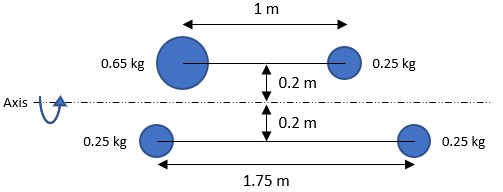## Mass Moments

For the point mass figure below, what is the mass moment of inertia?Hint
For point masses, the mass moment of inertia is:
$$I=\sum m_ir_i^2$$$where $$m$$ is the mass, and $$r$$ is the perpendicular distance to the rotation axis. Hint 2 Ignore the 1.75 m and 1 m distances. Those dimensions are there to throw you off. For point masses, the mass moment of inertia is: $$I=\sum m_ir_i^2$$$
where $$m$$ is the mass, and $$r$$ is the perpendicular distance to the rotation axis.
The figure has four point masses:
$$I=m_1r_{1}^{2}+m_2r_{2}^{2}+m_3r_{3}^{2}+m_4r_{4}^{2}$$$Remember, $$r$$ is the perpendicular distance the mass is from the rotation axis, not parallel distance, meaning the 1.75 m and 1 m dimensions are there for confusion and can be ignored. Starting from the bottom right point mass and moving clockwise: $$I=(0.25kg)(0.2m)^{2}+(0.25kg)(0.2m)^{2}+(0.65kg)(0.2m)^{2}+(0.25kg)(0.2m)^{2}$$$
$$=(0.04m^{2})[0.25kg+0.25kg+0.65kg+0.25kg]=0.056\:kg\cdot m^2$$$$$0.056\:kg\cdot m^2$$$